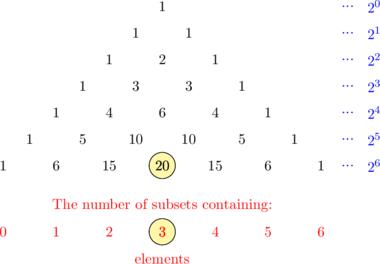# Subset

## Basic Concept

##### Subset and Proper Subset

$$\text{A}$$ is a subset of $$\text{A}$$ ($$\text{A}\subseteq \text{B}$$) if and only if every element of $$\text{A}$$ is in $$\text{B}$$

$$\text{A}$$ is a proper subset of $$\text{A}$$ ($$\text{A} \subset \text{B}$$) if and only if every element in $$\text{A}$$ is also in $$\text{B}$$,

and there exists at least one element in $$\text{B}$$ that is not in $$\text{A}$$

Example 1:

$$\text{A} = \lbrace 1, 2, 3 \rbrace$$

$$\text{B} = \lbrace 3, 1, 2 \rbrace$$

$$\text{A}$$ is a subset of $$\text{A}$$. ($$\text{A} \subseteq \text{B}$$)

Example 2:

$$\text{P} = \lbrace 5, 10, 15, 20 \rbrace$$

$$\text{Q} = \lbrace 5, 10, 15, 20, 25, 30 \rbrace$$

$$\text{P}$$ is a proper subset of $$\text{Q}$$ because the element 25 and 30 is not in the set $$\text{P}$$. ($$\text{P} \subset \text{Q}$$)

The empty set is therefore a proper subset of any non empty set

##### Total Number of Subsets

The total number of subsets is the number of sets with 0 elements, 1 elements, 2 elements, etc

The total number of subsets of a set with n elements is $$2^{n}$$

Example 1:

From the set below, list all the subsets and find the total number of subsets

$$\text{Q} = \lbrace \text{a, b, c, d}\rbrace$$

Subsets of $$\text{Q}$$ are:

$$\lbrace \rbrace,\:\:\lbrace \text{a}\rbrace, \:\: \lbrace \text{b}\rbrace, \:\: \lbrace \text{c}\rbrace, \:\: \lbrace \text{d}\rbrace\, \:\: \lbrace \text{a,b}\rbrace, \:\: \lbrace \text{a, c}\rbrace$$

$$\lbrace \text{a, d}\rbrace,\:\:\lbrace \text{b, c}\rbrace, \:\: \lbrace \text{b, d}\rbrace, \:\: \lbrace \text{c, d}\rbrace, \:\:\lbrace \text{a, b, c}\rbrace, \:\: \lbrace \text{a, b, d}\rbrace\, \:\: \lbrace \text{b, c ,d}\rbrace, \:\:\lbrace \text{a, c, d} \rbrace, \:\: \lbrace \text{a, b, c, d}\rbrace$$

Total number of subsets is $$2^n = 2^4 = 16$$

Example 2:

Find the number of subsets of  $$\text{P} = \lbrace \text{a, b, c, d, e, f}\rbrace$$  that contain exactly 3 elements

The total number of subsets of $$\text{P}$$ is $$2^{6}$$

Using Pascal's triangle:So, the number of subsets of $$\text{P}$$ that contain exactly 3 elements is 20